﻿ 超临界二氧化碳布雷顿循环热力学优化研究

# 超临界二氧化碳布雷顿循环热力学优化研究Investigations on Thermodynamic Optimization of Supercritical CO2 Brayton Cycle

Abstract: Supercritical carbon dioxide Brayton cycle is an important candidate in nuclear energy innovation. In this paper, an investigation on thermodynamic analysis and optimization was carried out for supercritical carbon dioxide Brayton cycles on the basis of the first law of thermodynamics. A new cycle, called double flow split compression cycle, was proposed to further increase system efficiency. Thermodynamic analysis and evaluation have been conducted. The effect of inter-cooling and re-heating on cycle efficiency has been obtained. A comparison of cycle efficiency has been made between the newly proposed double flow split compression cycle and traditional recompression cycle. The results show that cycle efficiency of double flow split compression cycle is 1-2 percent higher than that of recompression cycle. The efficiency improvement can exceed 3 percent while inter-cooling and re-heating were adopted in system.

1. 引言

2. 基本模型

2.1. 计算模型

${P}_{co}={P}_{ci}\cdot {\epsilon }_{c}$ (1)

${s}_{co}=s\left({P}_{ci},{T}_{ci}\right)$ (2)

${h}_{co}=h\left({P}_{co},{s}_{co}\right)$ (3)

$\Delta {h}_{c}=\left({h}_{co}-{h}_{ci}\right)/{\alpha }_{c}$ (4)

${T}_{co}=T\left[{P}_{co},\left({h}_{ci}+\Delta {h}_{c}\right)\right]$ (5)

${P}_{to}={P}_{ti}/{\epsilon }_{t}$ (6)

${s}_{to}=s\left({P}_{ti},{T}_{ti}\right)$ (7)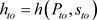(8)

$\Delta {h}_{t}=\left({h}_{to}-{h}_{ti}\right)\cdot {\alpha }_{t}$ (9)(10)

${W}_{t}={m}_{t}\cdot \Delta {h}_{t}$ (11)

${W}_{c}={m}_{c}\cdot \Delta {h}_{c}$ (12)

$\eta =\frac{{W}_{t}-{W}_{c}}{Q}$ (13)

2.2. 初始条件及参数

3. 计算结果分析

3.1. 再压缩循环计算验证Table 1. Initial conditions and equipment parameters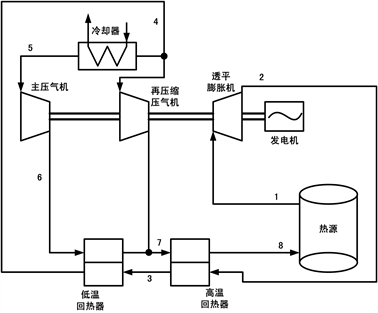Figure 1. Recompression cycle layoutTable 2. Calculation results comparison and model validation

3.2. 循环优化研究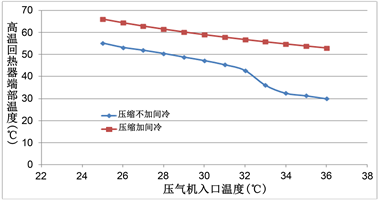Figure 2. Temperature difference at the hot end of high temperature recuperatorFigure 3. Double flow split compression cycle layout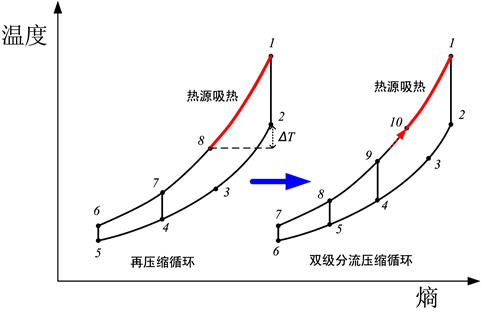Figure 4. T-S diagram of double flow split compression cycle

3.3. 双级分流压缩循环热力学特性研究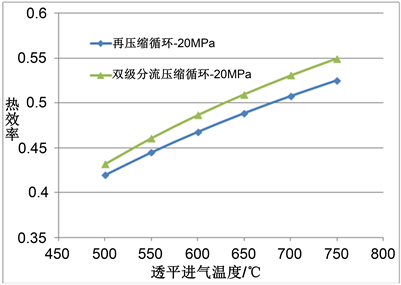Figure 5. Efficiency comparisons between double flow split compression cycle and recompression cycle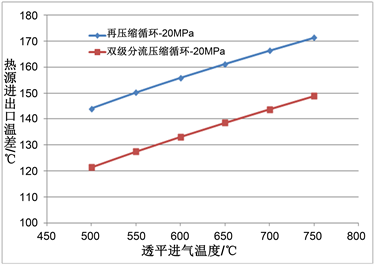Figure 6. Temperature rise comparisons of heat source between two cyclesTable 3. Comparisons of calculated efficiency in different cases

4. 结论

1) 传统再压缩循环仍存在较大的回热器端部温差，降低了热源平均吸热温度，系统热效率仍有进一步提升空间。本文在再压缩循环的基础上，提出了双级分流压缩循环，通过两次分流压缩，进一步降低了回热器端部温差，提高了热源平均吸热温度，进一步提升了超临界二氧化碳布雷顿循环的转换效率。

2) 在500℃~750℃的透平进气温度范围内，本文提出的双级分流压缩循环比传统再压缩循环的热效率高1~2个百分点，热源进出口温差降低20℃以上，且透平进气温度越高，效率优势越明显。当系统采用主压缩间冷和一级再热过程后，双级分流压缩循环比再压缩循环的热效率可高出3个百分点左右。

 Dostal, V., Driscoll, M.J. and Hejzlar. P. (2004) A Supercritical Carbon Dioxide Cycle for Next Generation Nuclear Reactors. Advanced Nuclear Power Technology Program, MIT-ANP-TR-100.

 黄彦平, 王俊峰. 超临界二氧化碳在核反应堆中的应用[J]. 核动力工程, 2012, 33(3): 21-27.

 Ahn, Y., Bae, S.J., Kim, M., Cho, S.K., Baik, S., Lee, J.I. and Cha, J.E. (2015) Review of Supercritical CO2 Power Cycle Technology and Current Status of Research and Development. Nuclear Engineering and Technology, 47, 647-661.
https://doi.org/10.1016/j.net.2015.06.009

 Delimont, J., McClung, A. and Portnoff, M. (2017) Direct Fired Oxy-Fuel Combustor for sCO2 Power Cycles: 1MW Scale Design and Preliminary Bench Top Testing. Proceedings of ASME Turbo Expo 2017, Charlotte, June 26-30 2017, Paper ID: GT2017-64952.
https://doi.org/10.1115/GT2017-64952

 Anselmi, E., Bunce, I., Pachidis, V., Zachos, P. and Johnston, M. (2018) An Overview of the Rolls-Royce sCO2-Test Rig Project at Cranfield. Proceedings of the Supercritical CO2 Power Cycle Symposium, Pittsburge, March 2018, Paper ID:049.

 Dostal, V. and Kulhanek. M. (2009) Research on Supercritical Carbon Dioxide Cycles in the Czech Republic. Proceedings of the Supercritical CO2 Power Cycle Symposium, Troy, April 2009.

 Lemmon, E.W., Huber, M.L. and McLinden. M.O. (2010) NIST Standard Refer-ence Database 23: Reference Fluid Thermodynamic and Transport Properties. REFPROP, Gaithersburg, Version 9.0.

 Zada, K.R., Kim, R., Wildberger, A. and Schalansky, C.P. (2018) Analysis of Supercritical CO2 Brayton Cycle Recuperative Heat Exchanger Size and Capital Cost with Variation of Layout Design. Proceedings of the Su-percritical CO2 Power Cycle Symposium, Pittsburg, March 2018, Paper ID: 165.

Top